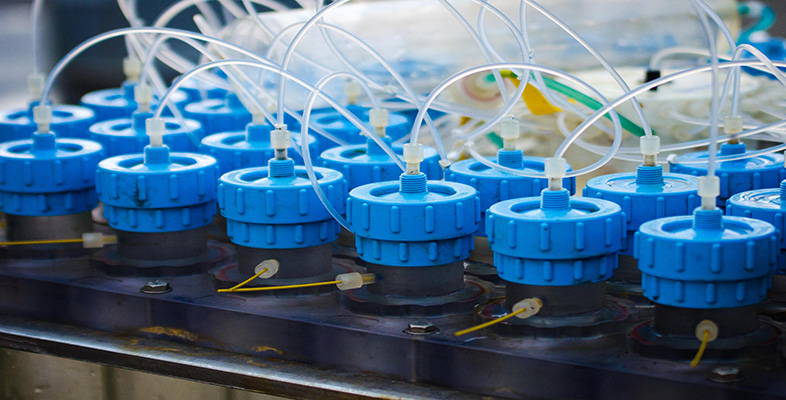Test kits for water analysis

Start this free course now. Just create an account and sign in. Enrol and complete the course for a free statement of participation or digital badge if available.

Free course

# Glossary

Accuracy
An expression of how close a given measurement from a set of results is to the true or accepted value.
Anode
The electrode in an electrochemical cell where oxidation takes place.
Calibration
Set of operations that establish, under specified conditions, the relationship between values indicated by a measuring instrument or measuring system and the corresponding known values of a standard.
Cathode
The electrode in an electrochemical cell where reduction takes place.
Digestion
Dissolving the whole of a sample, usually in acid, to destroy organic material.
Dynamic equilibrium
In a reversible chemical reaction the rate of the forward and reverse reactions are equal, so there is no net change in the system and the reaction is said to be at a point of dynamic equilibrium.
Electrical conductivity
Measure of the ability of a substance to conduct electric current.
Electrode
A component of an electrochemical cell at which oxidation or reduction takes place.
Electrochemical cell
A solution (the electrolyte) into which is immersed two electrodes which are in electrical contact with each other.
End-point
The concentration ratio of reactants where an indicator changes colour in a titration.
Equivalence point
In a titration, the point where reactants have reacted together in an exact ratio of quantities determined by the balanced chemical equation for the reaction (i.e. stoichiometrically).
Gravimetric analysis
A chemical analysis in which a desired constituent is converted into a substance (of known composition) that can be separated from the sample and weighed.
Interference
Concomitants in a sample that contribute to the analytical response.
Light scattering
Deviation of light from a straight path due to interactions with the medium through which it passes.
Oxidising agent
A substance that gains electrons during a redox chemical reaction
pH
The pH of an aqueous solution is an expression of its acidic or alkaline nature, and is defined as: pH = - log [H+ (aq)]
Precision
An expression of how reproducible a given measurement is.
Reducing agent
A substance that loses electrons during a redox chemical reaction
Robustness
The capacity of an analytical method to remain unaffected by small but deliberate variations in method parameters. This provides an indication of the method's reliability during normal usage.
Semi-quantitative
Falls short of the accuracy of a quantitative measurement (gives an approximation of a particular quantity).
Titration
The reaction of a solution of known concentration with a solution of unknown concentration until reaction is complete.
Working range
Concentration range over which the analytical method produces accurate quantitative data.
S230_1